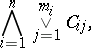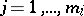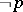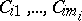# Conjunctive normal form

(diff) ← Older revision | Latest revision (diff) | Newer revision → (diff)

A propositional formula of the form(*)

where each,;, is either an atomic formula (a variable or constant) or the negation of an atomic formula. The conjunctive normal form (*) is a tautology if and only if for anyone can find both formulasandamong the, for some atomic formula. Given any propositional formula, one can construct a conjunctive normal formequivalent to it and containing the same variables and constants as. Thisis called the conjunctive normal form of.